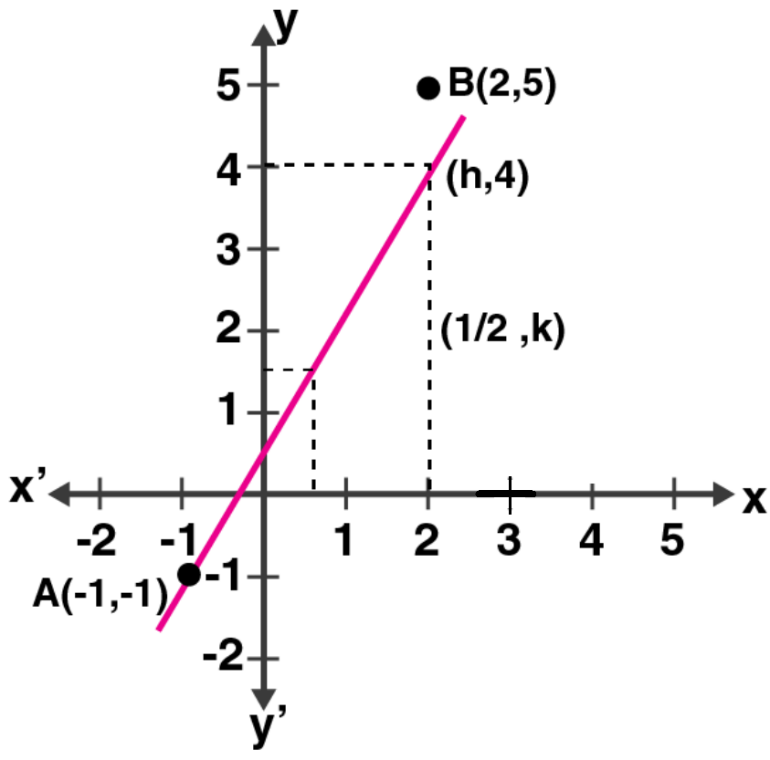Newbie

# Use a graph paper for this question. The graph of a linear equation in x and y, passes through A (– 1, – 1) and B (2, 5). From your graph, find the values of h and k, if the line passes through (h, 4) and 1/2, k).

• 0

This question is Based on Equation of a Straight Line Chapter of M.L Aggarwal book for ICSE BOARD for class 10.
Here you have to use graph paper for this question
The graph of a linear equation in x and y, passes through A (– 1, – 1) and B (2, 5).
From your graph, find the values of h and k, if the line passes through (h, 4) and (1/2, k).
This is the Question Number 21, Exercise 12.1 of M.L Aggarwal.

Share

1.Given,

Points (h, 4) and (1/2, k) lie on the line passing through A (-1, -1) and B (2, 5)From the graph, its clearly seen that

h = 3/2 and

k = 2

• -11